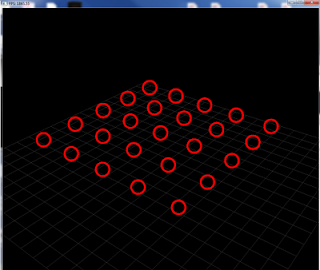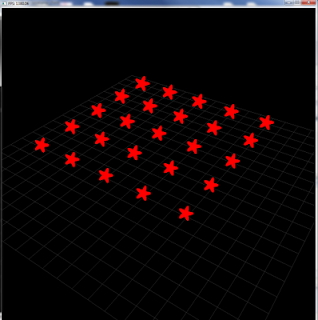## Thursday, December 30, 2010

### Circular and arbitrary shaped point sprites in opengl 3.3

I recently tried to convert an old OpenGL source of mine OpenGL 2.1 i guess to OpenGL 3.3 based on the new core profile. To my surprise some of the functions like glEnable(GL_POINT_SMOOTH) are deprecated in the core profile and it generates an error. That meant I could not use the built-in facility to render smooth anti-aliased roudned points. I was looking out for solutions and then I figured out a simple way of doing it using the fragment shader. In OpenGL 3.0 and above, you can use the gl_PointCoord variable to obtain the point's uv coordinates (which are in range of 0-1). This variable is what I needed. To generate the circular point sprite, you can do the following

` #version 330smooth out vec4 vFragColor;uniform vec4 vColor;void main() {   if(dot(gl_PointCoord-0.5,gl_PointCoord-0.5)>0.25)    discard; else   vFragColor = vColor;  }`

This gives the following outputWhich basically offsets the uv coordinates and then solves to see if the sqrt of distance is equal to 0.5 the radius of our point. I squre both sides to get the expression i have used. Similarly, a lot of interesting shapes could be generated as i show below.

Parametric coordinates:
We can use the parametric coordinate system (http://en.wikipedia.org/wiki/Polar_coordinate_system) to generate a lot of neat shapes of particles as I show below. For the polar coordinates, we need two things, radius and angle theta,
` vec2 p = gl_PointCoord* 2.0 - vec2(1.0);float r = sqrt(dot(p,p));`

For theta, we use
` float theta = atan(p.y,p.x);`

Now I show u how to generate a lot of neat shapes based on the polar coordinate expressions on the wikipedia page linked above,
Polar rose:
` if(dot(p,p) > cos(theta*5))  discard;else   vFragColor = vColor;`

This gives the following output,Round ring:
` if(dot(p,p) > r || dot(p,p) < r*0.75)  discard;else   vFragColor = vColor;`

You can change the multiplier to change the hole size.
This gives the following output,Spiral:
` if(dot(p,p)> 5.0/cos(theta-20*r))  discard;else   vFragColor = vColor;`

This gives the following output,Rounded star:
` if(dot(p,p) > 0.5*(exp(cos(theta*5)*0.75)) )  discard;else   vFragColor = vColor;`

This gives the following output,There might be other simpler ways of generating these shapes but these are some simpler ways to generate the various point sprite shapes.

Happy OpenGL coding.
Mobeen